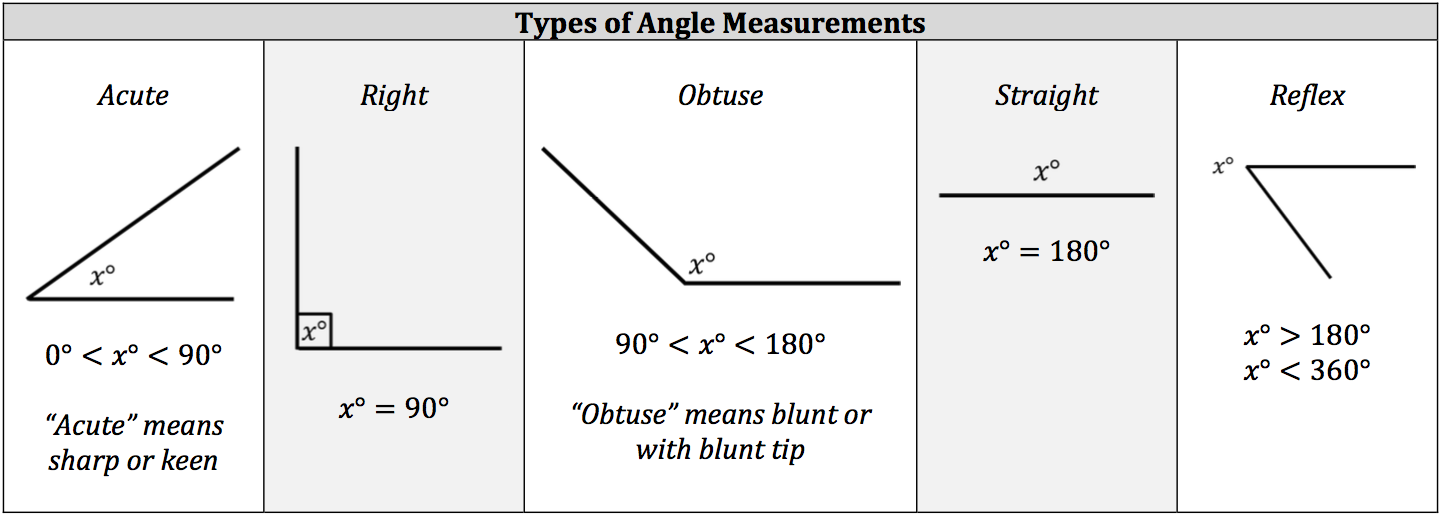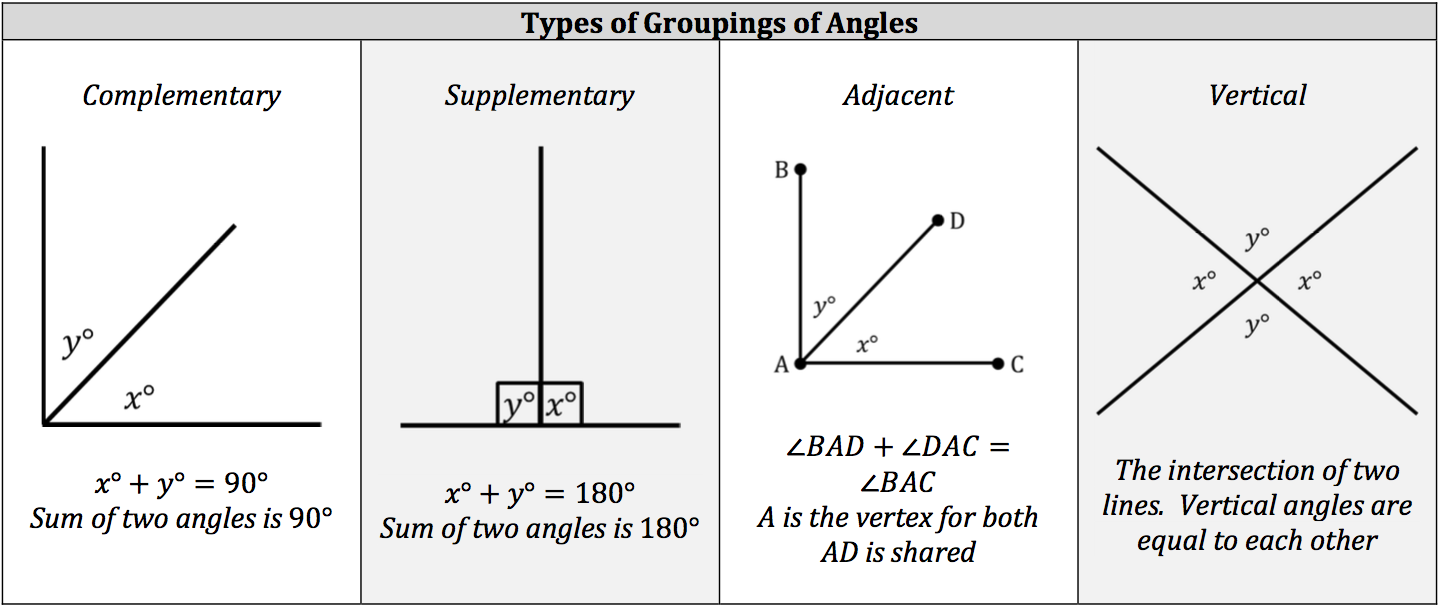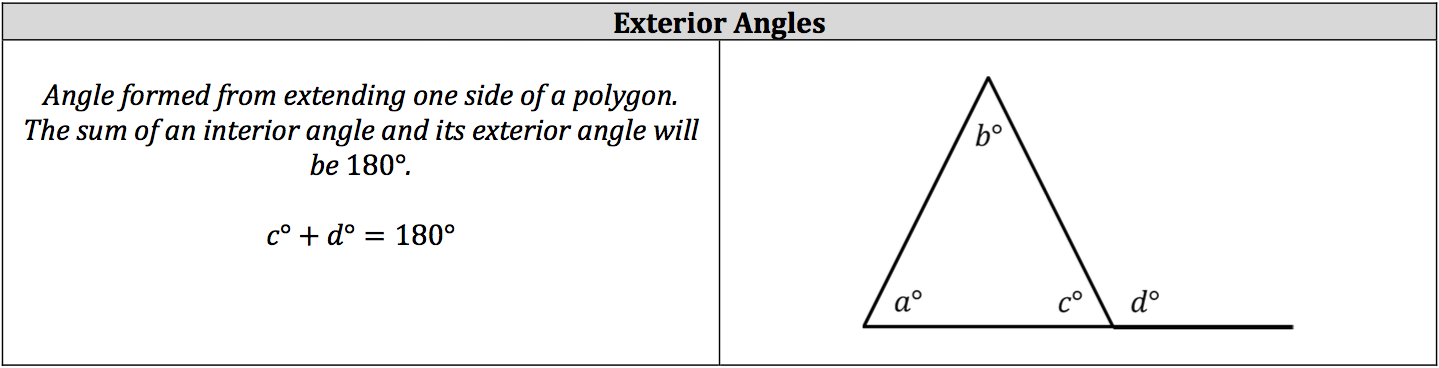Geometry is the branch of mathematics that encompasses the properties and relationships of shapes, such as points, angles, and solid figures. Like every other branch of mathematics, Geometry comes with its own set of notations.

## Types of Angles

An angle is formed when two rays share the same endpoint, which is called the vertex of the angle. In geometric notation, the vertex is the middle letter in the name of the angle. Each angle has a degree value, which is the measurement of the size of the angle, and the degree value determines its angle type. Groupings of angles are labeled by the sum of their angles or how they are formed.